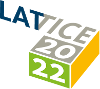#The 39th International Symposium on Lattice Field Theory (Lattice 2022)

Aug 8 – 13, 2022
Hörsaalzentrum Poppelsdorf
Europe/Berlin timezone

## Finite temperature QCD phase transition with 3 flavors of Mobius domain wall fermions

Aug 9, 2022, 2:20 PM
20m
CP1-HSZ/0.011 (CP1-HSZ) - HS1 (CP1-HSZ)

### CP1-HSZ/0.011 (CP1-HSZ) - HS1

#### CP1-HSZ

450
Show room on map
Oral Presentation QCD at Non-zero Temperature

### Speaker

Yu Zhang (RIKEN Center for Computational Science)

### Description

We investigate the phase structure of QCD with three degenerate quark flavors at finite temperature using Mobius domain wall fermions. To locate the critical endpoint and explore the order of phase transition on the diagonal line of the Columbia plot, we performed simulations at temperatures 131 and 196 MeV with lattice spacing $a\sim 0.12$ fm corresponding to temporal lattice extent $N_{\tau}=8,12$ with varying quark mass for two different volumes with aspect ratios $N_{\sigma}/N_{\tau}$ ranging from 2 to 3. By analyzing the volume and mass dependence of the chiral condensate, disconnected chiral susceptibility and Binder cumulant we find that there is a crossover at $m_q^{\mathrm{\overline {MS}}}(2\, \mathrm{GeV}) \sim 40\, \mathrm{MeV}$ for $\mathrm{T_{pc}}\sim$ 196 MeV and a transition point at $m_q^{\mathrm{\overline {MS}}}(2\, \mathrm{GeV}) \sim 4\, \mathrm{MeV}$ for $\mathrm{T}\sim$131 MeV on $24^3\times 12$ lattices. The $36^3\times12$ lattices are being investigated for the finite size scaling, we will present its result and discuss the nature of transition for $\mathrm{T}\sim$131 MeV.

### Primary author

Yu Zhang (RIKEN Center for Computational Science)

### Co-authors

Issaku Kanamori (RIKEN) Shoji Hashimoto (KEK, SOKENDAI) Takashi Kaneko (KEK) Yasumichi Aoki (RIKEN) Yoshifumi Nakamura (RIKEN)